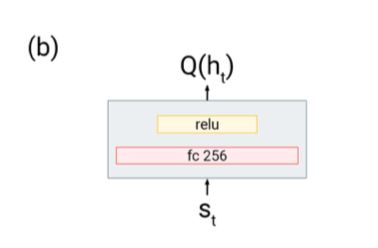(By DeepMind)

## Challenges

In this paper they focus on three challenges:

1. Sparse rewards
2. Partial observability
3. Random starting configurations

## Approach

Their approach is to use a version of off-policy, recurrent Q-learning.

• This allows them to outperform demonstrations
• The demo-ratio parameter controls the proportion of demonstrations vs agent experience, which greatly affects performance.

## The agent

The agent, referred as Recurrent Replay Distributed DQN from Demonstrations (R2D3), is designed to target all three challenges. Here's the architecture for the agent.

There are many actor processes, each of which is running the environment and its independent agent, in an interaction loop. Each actor streams its collected experience to a shared agent replay buffer.

A second demo replay buffer contains the demonstrations of the task.

From both replay buffers, trajectories are sampled with prioritization using a mix of max and mean of the TD-errors 1.

Next, the demo-ratio $$\rho$$ controls the proportion of data coming from each buffer 2.

Finally, the learned is trained with the prioritized training batch, using n-step, double Q learning, and a dueling architecture. The updated learned has to update the priorities of each trajectory in both buffers, as well as updating the weights of each actor.

Each buffer contains fixed-length ($$m=80$$) sequences of $$(s,a,r)$$ tuples, and each adjacent sequences overlap by 40 steps 3. Because of the recurrence, it would be best to start each sequence from the beginning of the episode, but a faster approximation would be to just replay the first 40 steps as a "burn-in" phase, and only train on the last 40 steps.

There are eight tasks that have been created.

Each of them are suitable to the three challenges of this paper, because they involve multiple high-level steps (sparse), in first-person (partially observed) and randomly generated (random initial conditions).

## Baselines

They compared the R2D3 agent with other baselines and ablations:• BC - for fair comparison the BC agent is trained with the same recurrent head as in the R2D3 agent.• DQfD No recurrence on R2D3, and replace with a FC layer 4.
• R2D2 No demonstrations on R2D3 ($$\rho=0$$)

## Experiments

### Training

Each type of agent was trained using 256 epsilon-greedy CPU-based actor processes, and 1 GPU-based learner process. The $$i$$-th actor is assigned a noise parameter $$\epsilon_i \in [0.4^8, 0.4]$$. Each agent was trained for at least 10 billion actor steps. BC was trained for 500k learner steps.

Each agent has an action-repeat factor of 2 - the actions received by the environment are repeated twice before obtaining the observation to the agent 5.

For all agents the network architecture is fed through a ResNet, then the output is augmented by concatenating the previous action $$a_{t-1}$$, previous reward $$r_{t-1}$$, and proprioceptive features $$f_t$$ (stateful observations like an object in hand). With the exception of DQfD, all agents use the same recurrent head which estimates the Q-value of a given state.

### Results

R2D3 is able to nontrivially learn 6 tasks, and exceed human performance on 3. No other baselines is able to learn anything.

The two unsolved tasks (Remember Sensor, Throw Across), have the most memory requirement, and because the burn-in is only 40 timesteps long, may not have enough context to complete the tasks.

On Wall Sensor, the agent learns to exploit the environment by tricking the sensor to stay active.

### Demo Ratio

On each tractable task, four different demo ratios were tested.

It appears that the lower the demo ratio the better (i.e., sampling very little from the expert demonstrations!)

### Guided exploration

In the Push Block task, R2D3 appears to have higher quality exploration, compared to that of R2D2. In the above figure (a), R2D3 concentrates on the center of the map. In (b), R2D3 is shown on average to push crates significantly more than R2D2. In (c), one example of R2D3 successfully copleting the task.

In the Baseball task, R2D3 is able to solve multiple subgoals (manually identified). At 4B steps, three R2D3 are able to consistently complete the task by completing all of the required subgoals.

1. $$\eta=1.0$$ as per https://arxiv.org/pdf/1803.00933.pdf [return]
2. For each element in the training batch, randomly sample from the demo replay buffer with probability $$\rho$$, otherwise sample from the agent replay buffer. [return]
3. Sequences don't cross episode boundaries, so the environment will not reset in the middle of a sequence. [return]
4. Not restricted to demo-ratio $$\rho=0.25$$, as in DQfD [return]
5. On Atari, 4 repeated actions are common but it makes it really difficult for the demonstrators (when collecting demonstration data). 2 is a compromise. [return]